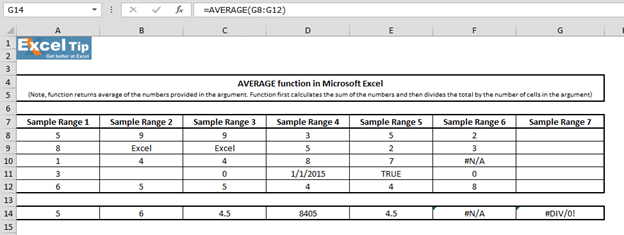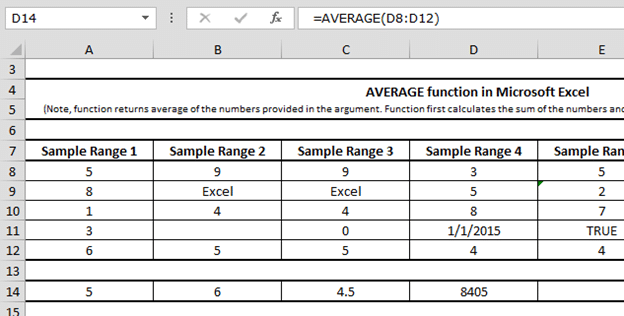# The AVERAGE function in Excel is one of the most common statistical functions. This function calculates the mean (arithmetic mean) of the numbers given in the argument. AVERAGE is calculated by adding the number of cells and then dividing by the total number of cells in the argument.

To use the Average function in Excel software, refer to the 7 examples given here: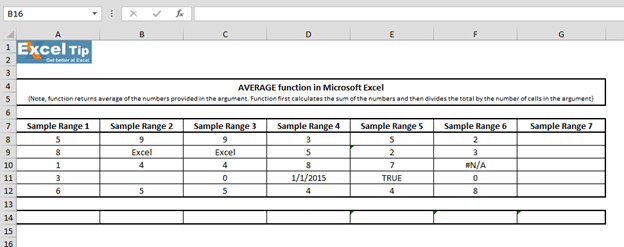### Example 1:

In the sample1 column we have a small number of numbers that we want to calculate their average. Follow these steps:

• Enter the AVERAGE function in cell A14

= AVERAGE (A8: A12,7)

• Press Enter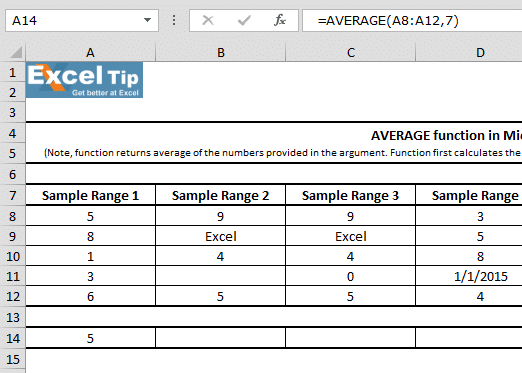Note : You can put up to 255 arguments in the 2007 or later versions of Excel.

Explanation of the formula: Consider A8 to A12 as the range of our first argument, then close the parentheses and hit enter . This function returns 5 as the average of 5 numbers. In other words, the sum of the numbers 5 + 8 + 1 + 3 + 6 will be equal to 23. And as you can see, we have 5 cells in the argument, so we have to divide 23 by 5. The answer is about 5, which is the average.

### Second example:

In this example, we will see how the AVERAGE function treats empty cells and cells that contain text. Follow these steps to understand this:

• Enter the AVERAGE function in cell B14
• Then select B8: B12 as the range

= AVERAGE (B8: B12)

• Press Enter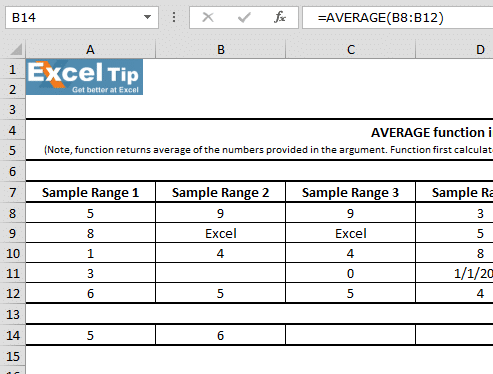Explanation of the formula: In this case, the function returns the number 6 as the average. As you can see, cell B9 is ignored by the AVERAGE function (contains text) as well as cell B11 which is empty.

### Third example:

In this example, we will see how the function deals with cells that have a value of zero. Follow these steps:

• Enter the function in cell C14
• Groups of C8 to C12 choose

= AVERAGE (C8: C12)

• Press Enter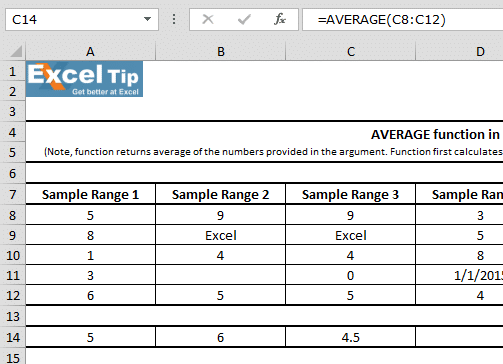### Example 4:

In this example, we see that one of the cells has a date. So in this example we will see how the mean function works in dealing with history.

• Enter the function in cell D14

= AVERAGE (D8: D12)

• Press Enter

The function returns the number 8405. This is because in Excel, dates are stored as numbers. In this example, January 1, 2015 is equivalent to the number 42,005.### Example 5:

In this example, we put a text in the range where we want to calculate its average.

• Enter the function in cell E14

= AVERAGE (E8: E12)

• Press Enter
• The function returns the number 4.5 as a result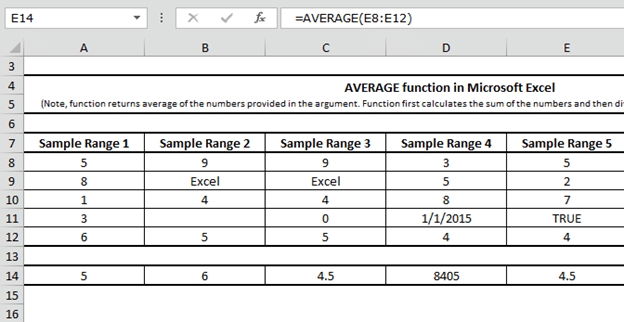This means that the Average function does not take the text into account when performing calculations, thus providing an accurate result.

### Example 6:

In this example, we learn how the Average function will work in the face of a cell with an #N / ​​A error. ( Na in the word not available means no access. When this error occurs, Excel Tells you that the desired value does not exist in the defined range.)

• Enter the function in cell F14

= AVERAGE (F8: F12)

• Press Enter
• Returns the # N / A function as a result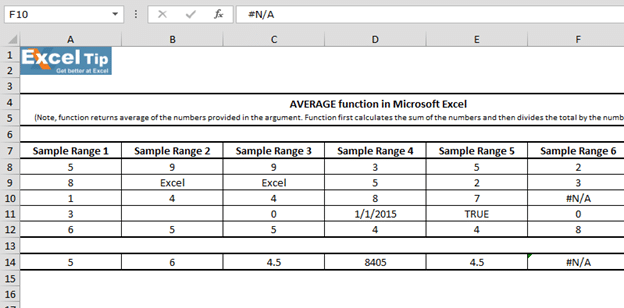If each cell contains an error, it returns the error function as a result.

### Example 7:

In this example, we will examine how the average function will work if the selected range is empty .